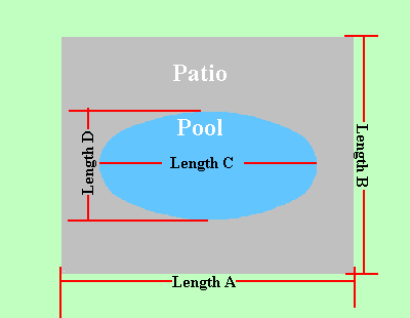Spike's Calculators

# Rectangular Shaped Concrete Slab - Oval Shaped Pool - Metric Measurements

Calculate the amount of concrete needed to pour a slab around an oval shaped pool using metric measurements.

Includes results in square metres for the patio area and the outer perimeter of this area in lineal metres. The square metres result of this patio area can be used to estimate different materials and the outer perimeter of the patio can be used to estimate the fencing material..### Rectangular Shaped Pool Slab - Oval Pool

Length A m
Length B m
Length C of Pool m
Length D of Pool m
Required Slab Depth mm

#### Results:

 Area of Pool m² Area of Pool Patio m² Outer Perimeter lin m Concrete Needed in Cubic Metres m³ Concrete Needed in Cubic Yards yd³

#### Calculations

1. the overall area length (length A in metres)
2. the overall area width (length B in metres)
3. length of pool (length C in metres)
4. width of pool (length D in metres)
5. depth of concrete slab (millimetres)

#### Results

1. square metres of the pool area
2. square metres of the slab area around the pool
3. perimeter of outer area (lineal metres)
4. concrete needed in cubic metres
5. concrete needed in cubic yards